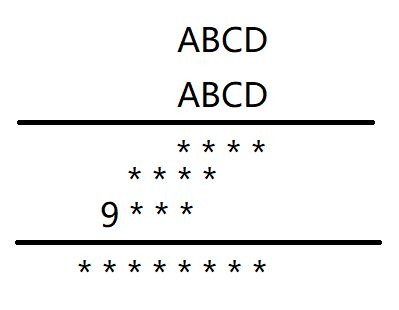# A,B,C,D分别为不同的整数，满足以下乘法公式，求A,B,C,D的值由题意可知A,B,C,D为不同的整数，则A!=B,A!=C,A!=D,B!=C,B!=D,C!=D

let count=3999     //因条件限制千位为3，则从3999开始
let a,b,c,d,e,f,fx,ex
for(let num=3000;num<count;num++){
d=num/1%10;                            //d表示num的个位数
c=parseInt(num/10%10);          //c表示num的十位数
b=parseInt(num/100%10);        //b表示num的百位数
a=parseInt(num/1000%10);    //a表示num的千位数

e=a*num      //e表示解题步骤第行的值
f=b*num    //f表示解题步骤第二行的值
g=d*num   //g表示解题步骤第一行的值
if(g.toString().length==4&&f.toString().length==4&&e.toString().length==4){
fx=parseInt(f/1000%10);   //fx表示解题步骤的第二行的千位数
let x=parseInt(e/1000%10);//e表示解题步骤的第三行的千位数9
ex=parseInt(e/100%10);//ex表示解题步骤的第三行的百位数
if(x==9){
if(a!=b&&a!=c&&a!=d&&b!=c&&b!=d&&c!=d&&c==0){
console.log('a=>'+a+' b=>'+b+' c=>'+c+' d=>'+d)
}

}
}
}
console.log('done')                        

PS D:\NodePack\Node_base\nodejsStudo> node .\zushu.js
a=>3 b=>2 c=>0 d=>1
done
PS D:\NodePack\Node_base\nodejsStudo> 

posted @ 2021-04-14 15:59  墨染暖栀  阅读(28)  评论(0编辑  收藏  举报
/* 看板娘 */ /* 粒子吸附*/